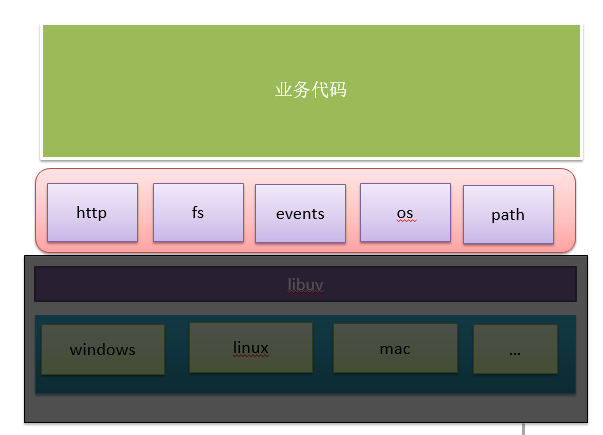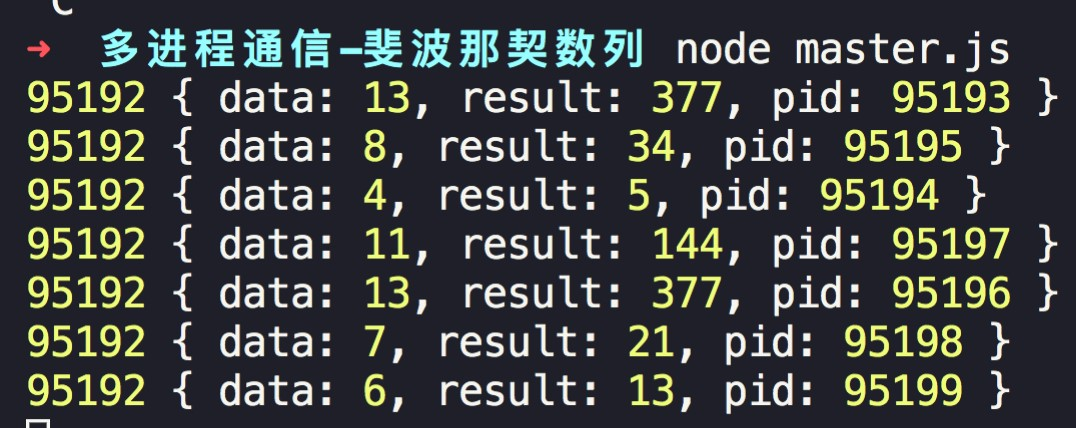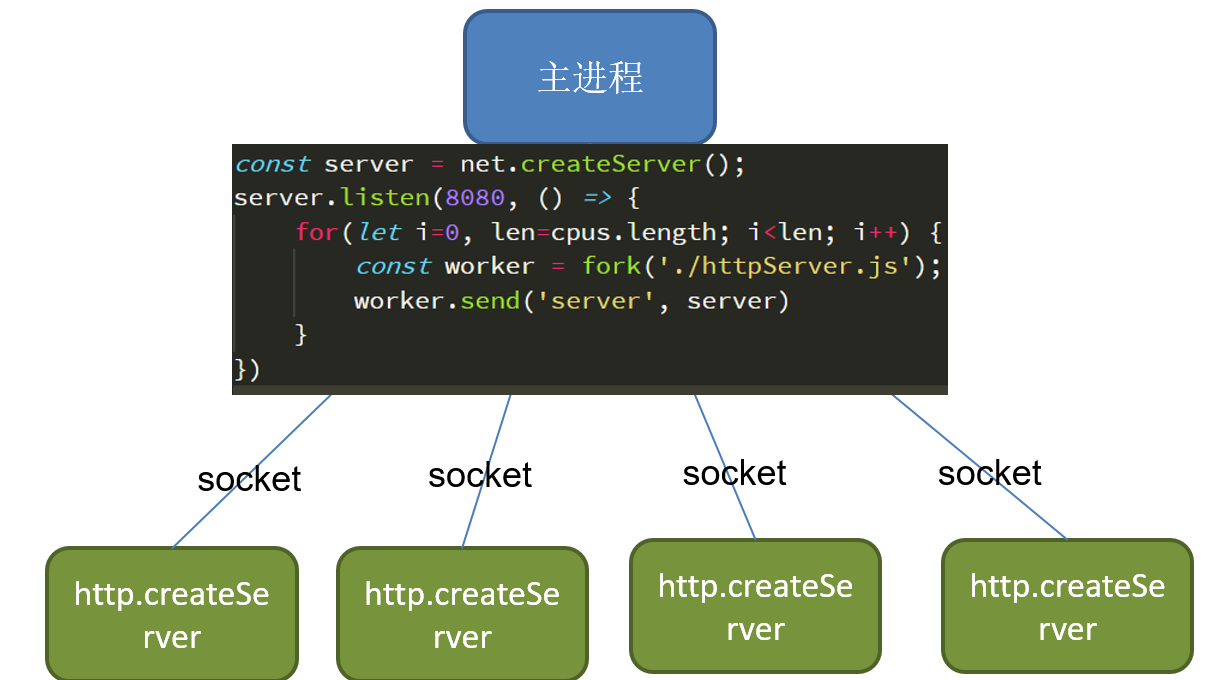node is single-threaded, how can our node project utilize the resources of a multi-core CPU while improving the stability of the node service?

2. node multi-process creation
3. multi-process communication
4. multi-process maintenance

### 1. single thread of node

A process is a dynamic execution of a program with certain independent functions on a dataset, an independent unit of resource allocation and scheduling by the operating system, and a vehicle for the operation of an application.

A thread is a single sequential control flow in program execution, which exists within a process, and is a smaller basic unit than a process that can run independently.

In the early days of single-core CPU systems, the concept of process was introduced to realize multitasking. Different programs ran in processes with isolated data and instructions, and were executed through time-slice rotation scheduling.

The system overhead is high because of the need to save relevant hardware site, process control blocks and other information during process switching. In order to further improve the system throughput and make fuller use of CPU resources while the same process is executing, the concept of threads is introduced. Threads are the smallest unit of OS scheduling execution, they are attached to the process, share the resources in the same process, and basically do not own or only own a small amount of system resources, so the switching overhead is very small.

Node is built on top of the V8 engine, which dictates a mechanism similar to that of a browser.

A node process can only utilize one core, and node can only run in a single thread. Strictly speaking, node is not really a single-threaded architecture, i.e., there can be multiple threads within a process, because node itself has certain i/o threads that exist, and these I/O threads are handled by the underlying libuv, but these threads are done transparently to the node developer, and are only used in C++ extensions. Here we will shield the underlying details and focus exclusively on what we want to focus on.The advantages of single-threaded are: single program state, no locking, thread synchronization problems in the absence of multiple threads, and the operating system can also improve CPU usage very well when scheduling because of less context switching. However, single-core single-threaded has corresponding disadvantages.

• the whole program hangs when this thread hangs.
• Inability to fully utilize multi-core resources.

### 2. node multi-process creation

node provides the `child_process` module, which provides several methods to create child processes.

 ``````1 `````` ``````const { spawn, exec, execFile, fork } = require('child_process'); ``````

All four methods can create subprocesses, but the way they are used is slightly different. Let’s take the example of creating a subprocess to calculate the Fibonacci series numbers, with the file of the subprocess (worker.js).

 `````` 1 2 3 4 5 6 7 8 9 10 11 12 13 14 15 16 17 `````` ``````// worker.js const fib = (num) => { if (num === 1 || num === 2) { return num; } let a = 1, b = 2, sum = 0; for (let i = 3; i <= num; i++) { sum = a + b; a = b; b = sum; } return sum; } const num = Math.floor(Math.random() * 10) + 3; const result = fib(num); console.log(num, result, process.pid); // process.pid表示当前的进程id ``````

How do you call these methods in master.js to create a child process?

Commands Use Explanation
spawn spawn(’node’, [‘worker.js’]) start a word process to execute a command
exec exec(’node worker.js’, (err, stdout, stderr) => {}) start a subprocess to execute the command, with callbacks
execFile exexexFile(‘worker.js’) start a subprocess to execute the executable(add # to the header! /usr/bin/env node)
fork fork(‘worker.js’) is similar to spawn, but here you only need to customize the js file module

Take the fork command as an example.

 ``````1 2 3 4 5 6 `````` ``````const { fork } = require('child_process'); const cpus = require('os').cpus(); for(let i=0, len=cpus.length; i

### 3. Communication between multiple processes

The communication between processes in node is mainly between master and slave (child) processes. The child processes cannot communicate with each other directly, and if they want to communicate with each other, they have to forward the information through the master process.

The master and child processes communicate with each other through IPC (Inter Process Communication), which is also implemented by the underlying libuv according to different operating systems.

Let’s take the example of calculating the Fibonacci sequence, where we use the number of cpu processes minus one to do the calculation and the remaining one to output the result. This requires the sub-process responsible for the calculation to pass the result to the main process, and then let the main process pass it to the output to perform the output. Here we need 3 files.

• master.js: to create the subprocesses and the communication between the subprocesses.
• fib.js: to calculate the Fibonacci sequence.
• log.js: to output the result of the Fibonacci series calculation.

Main process.

 `````` 1 2 3 4 5 6 7 8 9 10 11 12 13 14 `````` ``````// master.js const { fork } = require('child_process'); const cpus = require('os').cpus(); const logWorker = fork('./log.js'); for(let i=0, len=cpus.length-1; i { // 计算后返回的结果 logWorker.send(data); // 将结果发送给输出进程 }) } ``````

Calculation process.

 `````` 1 2 3 4 5 6 7 8 9 10 11 12 13 14 15 16 17 18 19 20 21 22 `````` ``````// fib.js const fib = (num) => { if (num===1 || num===2) { return num; } let a=1, b=2, sum=0; for(let i=3; i { const result = fib(num); process.send(JSON.stringify({ num, result, pid: process.pid })) }) ``````

Output process.

 ``````1 2 3 `````` ``````process.on('message', data => { console.log(process.pid, data); }) ``````

When we run master, we can see the results of the individual child process calculations.The first number indicates the number of the current output sub-process, followed by the data calculated in each sub-process.

Similarly, we can use a similar idea when logging the http service, where multiple subprocesses take on the http service and the remaining subprocesses do the logging and other operations.

When I want to create a server with subprocesses, I use the above Fibonacci sequence-like idea and change fib.js to httpServer.js.

 `````` 1 2 3 4 5 6 7 8 9 10 `````` ``````// httpServer.js const http = require('http'); http.createServer((req, res) => { res.writeHead(200, { 'Content-Type': 'text/html' }); res.end(Math.random()+''); }).listen(8080); console.log('http server has started at 8080, pid: '+process.pid); ``````

The result is an error, indicating that port 8080 is already occupied.

 ``````1 `````` ``````Error: listen EADDRINUSE: address already in use :::8080 ``````

This is because: there is a file descriptor for the socket socket listening port on the TCP side, and each process has a different file descriptor, which will fail when listening to the same port.

There are two solutions: the first and simplest is that each child process uses a different port, and the master process gives the cyclic identifier to the child process, which uses the relevant port by this identifier (e.g. the identifier passed in from 8080+ as the port number of the current process).

The second option is to do port listening in the master process and then pass the listening socket to the child process.Main process.

 `````` 1 2 3 4 5 6 7 8 9 10 11 12 13 `````` ``````// master.js const fork = require('child_process').fork; const net = require('net'); const server = net.createServer(); const child1 = fork('./httpServer1.js'); // random const child2 = fork('./httpServer2.js'); // now server.listen(8080, () => { child1.send('server', server); child2.send('server', server); server.close(); }) ``````

httpServer1.js:

 `````` 1 2 3 4 5 6 7 8 9 10 11 12 13 14 15 16 `````` ``````const http = require('http'); const server = http.createServer((req, res) => { res.writeHead(200, { 'Content-Type': 'text/plain' }); res.end(Math.random()+', at pid: ' + process.pid); }); process.on('message', (type, tcp) => { if (type==='server') { tcp.on('connection', socket => { server.emit('connection', socket) }) } }) ``````

httpServer2.js:

 `````` 1 2 3 4 5 6 7 8 9 10 11 12 13 14 15 16 `````` ``````const http = require('http'); const server = http.createServer((req, res) => { res.writeHead(200, { 'Content-Type': 'text/plain' }); res.end(Date.now()+', at pid: ' + process.pid); }); process.on('message', (type, tcp) => { if (type==='server') { tcp.on('connection', socket => { server.emit('connection', socket) }) } }) ``````

Our 2 servers, one outputting random numbers and the other outputting the current timestamp, can be found to be functioning properly. Also, because these process services are preemptive, whichever process grabs the connection will handle the request.

What we should also know is that.

The memory data between each process is not interoperable, so if we cache data in one process using a variable, another process cannot read it.

### 4. Multi-process guarding

The multiprocess we created in part 3 solves the problem of multi-core CPU utilization, and then we have to solve the problem of process stability.

Each child process will trigger the `exit event` when it exits, so we can listen to the exit event to know that a process has exited, and then we can create a new process to replace it.

 `````` 1 2 3 4 5 6 7 8 9 10 11 12 13 14 15 16 17 18 19 20 21 22 23 `````` ``````const fork = require('child_process').fork; const cpus = require('os').cpus(); const net = require('net'); const server = net.createServer(); const createServer = () => { const worker = fork('./httpServer.js'); worker.on('exit', () => { // 当有进程退出时，则创建一个新的进程 console.log('worker exit: ' + worker.pid); createServer(); }); worker.send('server', server); console.log('create worker: ' + worker.pid); } server.listen(8080, () => { for(let i=0, len=cpus.length; i

#### cluster module

For the multi-process daemon part, node has also introduced the `cluster module` to solve the multi-core CPU utilization problem. The cluster also provides exit events to listen to the exit of child processes.

A classic example.

 `````` 1 2 3 4 5 6 7 8 9 10 11 12 13 14 15 16 17 18 19 20 21 22 23 24 `````` ``````const cluster = require('cluster'); const http = require('http'); const cpus = require('os').cpus(); if (cluster.isMaster) { console.log(`主进程 \${process.pid} 正在运行`); // 衍生工作进程。 for (let i = 0, len=cpus.length; i < len; i++) { cluster.fork(); } cluster.on('exit', (worker) => { console.log(`工作进程 \${worker.process.pid} 已退出`); cluster.fork(); }); } else { http.createServer((req, res) => { res.writeHead(200); res.end(Math.random()+ ', at pid: ' + process.pid); }).listen(8080); console.log(`工作进程 \${process.pid} 已启动`); } ``````

### 5. Summary

Although node is single-threaded, we can make full use of multi-core CPU resources by creating multiple sub-processes, and we can improve the overall stability of our project by listening to some events of the process to sense the running status of each process.